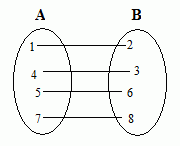Name: ___________________Date:___________________

 Email us to get an instant 20% discount on highly effective K-12 Math & English kwizNET Programs!

High School Mathematics - 24.11 Functions, Relations, Domain & Range

 Relation: A relation is simply a set of ordered pairs. The first elements in the ordered pairs, the x-values, form the domain. The second elements in the ordered pairs, the y-values, form the range.This mapping shows a relation from set A into set B. The relation consists of the ordered pairs (1,2), (1,3), (4,6), (5,6), and (7,8). The domain is the set {1, 4, 5, 7} The range is the set {2, 3, 6, 8}. The range is the dependent variable. Function: A function is a set of ordered pairs in which each element of the domain has only ONE element associated with it in the range. The relation shown above is NOT a function because the element 1 (in the domain) is mapped to two elements in the range, 2 and 3. A function may have two x-values assigned to the same y-value, but may not have two y-values assigned to the same x-value. ExampleDomain = {1,4,5,7} Range = {2,3,6,8} Relation = {(1,2), (4,3), (5,6), (7,8)} This relation is a function. Directions: Answer the following. Enclose the answer in curly brackets { }. Also write at least 5 examples of your own.
Q 1: Write the rule of the function:
 Input 0 1 4 6 10 Output 2 3 6 8 12

y = x + 2
y = 2x + 1
y = 2x

Q 2: The domain of the function y = 2x is 0,2,5,7, and 8. Find the range of the function.

Q 3: The domain of the function y = x + 3 is 0,1,2,3, and 4. Find the range.

Q 4: Is the relation a function? {(ñ3, 5), (ñ2, 5), (ñ1, 5), (0, 5), (1, 5), (2, 5)}
Not a function
Function

Q 5: State the domain of the following relation: {(2, ñ3), (4, 6), (3, ñ1), (6, 6), (2, 3)}

Q 6: Find the range of the function y = x - 5 given the domain {1,2,3,4}

Q 7: State the domain of the following relation: {(ñ3, 5), (ñ2, 5), (ñ1, 5), (0, 5), (1, 5), (2, 5)}

Q 8: State the range of the following relation. {(ñ3, 5), (ñ2, 5), (ñ1, 5), (0, 5), (1, 5), (2, 5)}

Question 9: This question is available to subscribers only!

Question 10: This question is available to subscribers only!